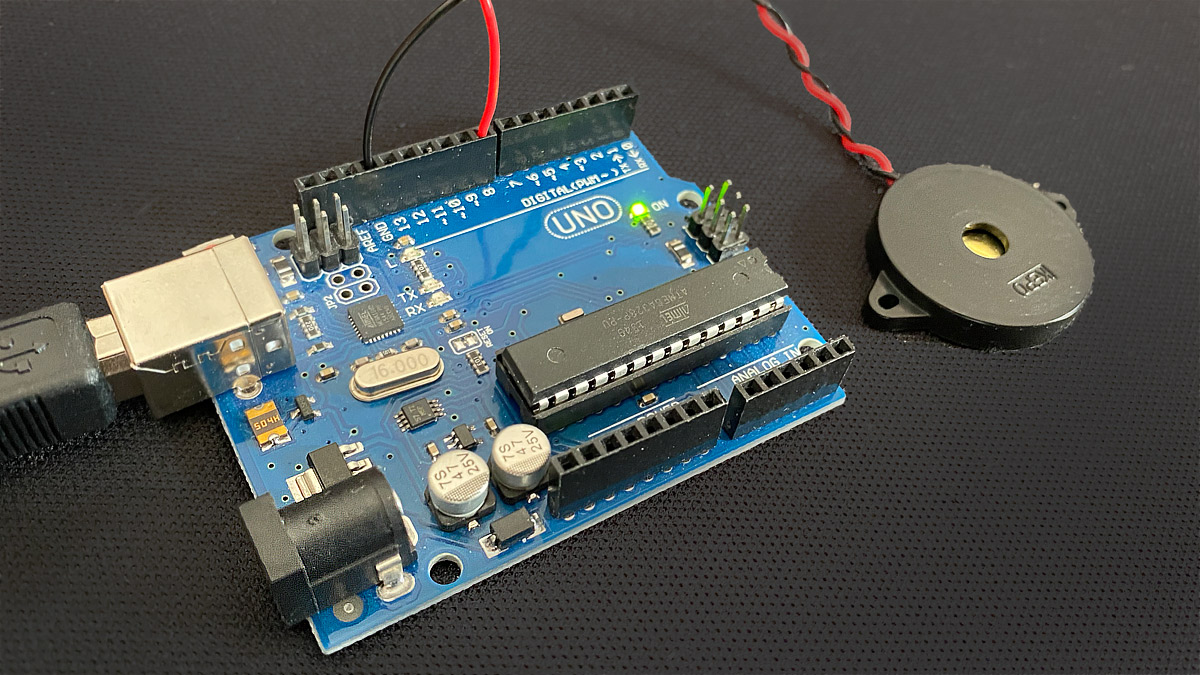# Morse code with ArduinoIf you dive into the world of microcontroller programming one of the first exercises is the flashing of an SOS in Morse code. But what happens then? Morse code with Arduino: In this project you’ll learn how to enter text via the serial monitor and convert it into blinking and beeping with Arduino.

## Components

• Arduino UNO*
• Piezo speaker* (Tip: You can also easily extract them from old melody greeting cards).

## Circuit: Morse code with Arduino

The circuit consists of an Arduino UNO and a piezo speaker. This is simply connected to digital pin 8 and the GND of the Arduino.

## Code: Morse code with Arduino

``````String inputString = "";        // stores incoming data
bool stringComplete = false;    // is true if the received string is complete
int ledPin = 13;                // Output Pin LED
int speakerPin = 8;             // Pin of the loudspeaker

static String morseLetters[] = {".-", "-...", "-.-...", "-...", "...", "...", "...", ".---", "-.---", ".-...", "--", "-...", "---", ".---", ".---", "--.---",
".-.", "...", "-", "..-", "...-", ".--", "-..-", "-.--", "--..", "E"
};
static String morseNumbers[] = {"-----", ".----", ".----", "...---", "...." , "-....", "--...", "---..", "----."};

void setup() {
Serial.begin(115200);           // start serial communication
inputString.reserve(200);       // creates a 200-byte buffer for incoming data
pinMode(ledPin, OUTPUT);        // declares ledPin as output
}

void loop() {
if (stringComplete) {           // if it was received completely
Serial.println(inputString);  // display it in the Serial Monitor
morseOutput(inputString);     // call morseOutput method and pass the string
inputString = "";             // Reset string
stringComplete = false;       // Set stringComplete to false until a string has been received completely
}
}

void serialEvent() {                    // is executed automatically after each pass of loop()
while (Serial.available()) {          // as long as serial data are available
char inChar = (char)Serial.read();  // reads the current byte
inputString += inChar;              // add the byte as Char (character) to the string inputString

if (inChar == '\n') {               // if the character is a line break, the reception of the data is completed
stringComplete = true;            // then set stringComplete to true so that the loop method can output the data
}
}
}

void morseOutput(String myString) {
// current letter is converted to integer (Ascii code), which results in e.g. for the small a 97
String currentLetter = "";                                          // stores the current Morse code for a letter between
for (int i = 0; i < myString.length(); i++) {                       // for 0 to length of the string
if ((int(myString[i]) >= 97) && (int(myString[i]) <= 122)) {      // if letter from a to z
currentLetter = morseLetters[int(myString[i]) - 97];            // currentLetter = position in Morse code array from 97
} else if ((int(myString[i]) >= 65) && (int(myString[i]) <= 90)) {// if letter from A to Z
currentLetter = morseLetters[int(myString[i]) - 65];              // currentLetter = position in Morse code array from 65
} else if ((int(myString[i]) >= 48) && (int(myString[i]) <= 57)) {// if number from 0 to 9
currentLetter = morseNumbers[int(myString[i]) - 48];              // currentLetter = position in Morse code number array from 48
} else {
currentLetter = " ";                                            // for all other characters, insert a space
}

Serial.print(currentLetter);                                      // display the Morse code in the serial monitor
Serial.print(" ");                                                // Space in Serial Monitor

for (int j = 0; j < currentLetter.length(); j++) {                // for 0 to length of current Letter
if (int(currentLetter[j]) == 46) {                                // check if current character is a dot (Ascii-Code 46)
digitalWrite(ledPin, HIGH);                                   // switch LED on
tone(speakerPin, 200);                                        // switch on loudspeaker, pitch 200 Hz
delay(50);                                                    // short pause
digitalWrite(ledPin, LOW);                                    // switch off LED
noTone(speakerPin);                                           // switch off speaker
delay(50);                                                    // short pause
} else if (int(currentLetter[j]) == 45) {                       // check if current character is a bar (Ascii-Code 45)
digitalWrite(ledPin, HIGH);                                   // switch LED on
tone(speakerPin, 200);                                        // switch on loudspeaker, pitch 200 Hz
delay(150);                                                   // longer pause
digitalWrite(ledPin, LOW);                                    // switch off LED
noTone(speakerPin);                                           // switch off speaker
delay(150);                                                   // longer pause
} else {
delay(150);                                                   // longer pause, character unknown
}
}
delay(200);                                                       // Pause between
}
}
``````

Translated with www.DeepL.com/Translator (free version)